## Tables index

#### Oliver Kasele, Lucien Momeka, Grace Bahaya, Bahati Ntumwa

Journal of Finance and Economics. 2019, 7(4), 148-163 doi:10.12691/jfe-7-4-5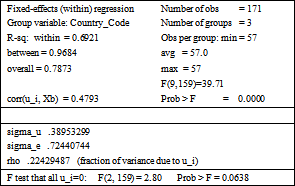• Table 1. Individual effects for the first model (INVPRI)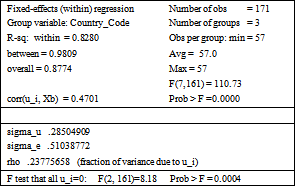• Table 2. Individual effects for the second model (INVPUB)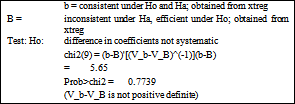• Table 3. First model Hausman test (INVPRI)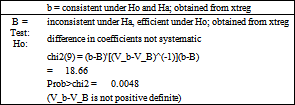• Table 4. Second model Hausman test (INVPUB)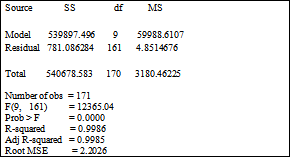• Table 5. First model Homoscedasticity test (INVPRI)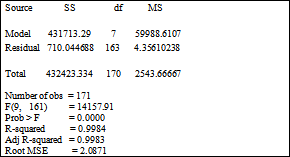• Table 6. Second model Homoscedasticity test (INVPUB)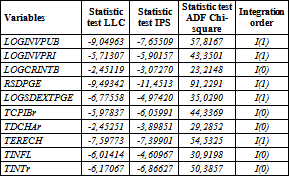• Table 7. LLC, IPS and ADF Chi-square unit test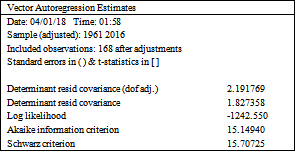• Table 8. Number of lags applied to the cointegration test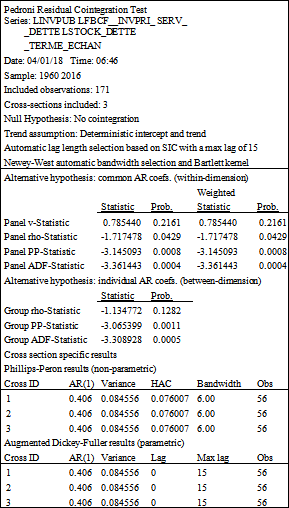• Table 9. Pedroni cointegration test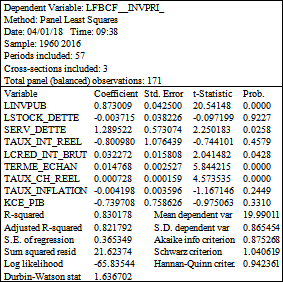• Table 10. First model Estimation of the short-term relationship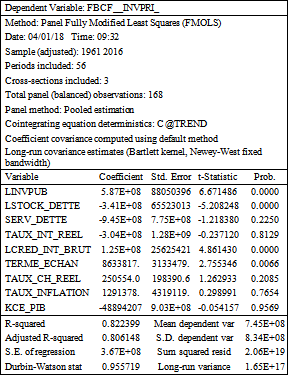• Table 11. First model Estimation of the long term relationship with FMOLS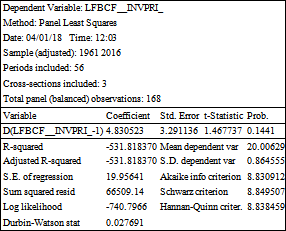• Table 12. Correction coefficient Estimation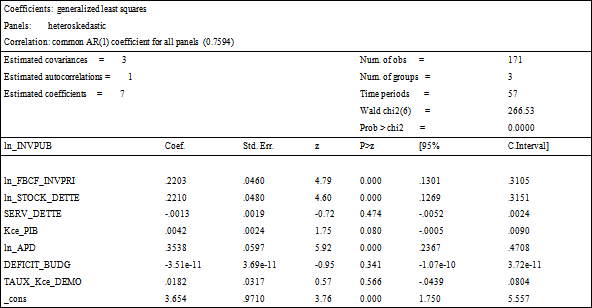• Table 13. Second model estimate with generalized least squares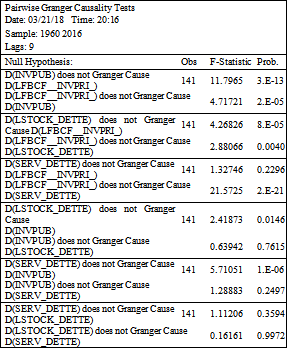• Table 14. Granger causality test